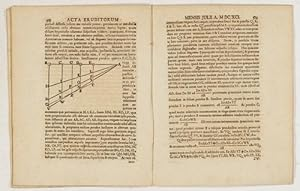## MCMC for conditional Bernoullis

Posted in Books, Statistics, University life with tags , , , , , on February 22, 2021 by xi'anJeremy Heng, Pierre Jacob [currently in Paris!] and Nianqiao Ju are in a recent arXival considering the simulation of a conditional Bernoulli, namely generating a vector of N Bernoullis with different probabilities under the constraint that their sum is fixed as I. Rather than going for a perfect simulator, with cost O(NI), they opt for the simplest of MCMC samplers, where a 0 and a 1 entries are exchanged at random. In connection with a recent spate of MCMC works using coupling, they establish convergence in O(N log N) steps, even when the probabilities are arbitrarily close to zero and one. Including the case when they are Uniformly generated. From a mundane perspective, I wonder at the appeal of using the probabilities to select the exchange pair. I realise sorting the probabilities is already of order O(N log N) avoiding selecting highly probable 1’s and highly probable 0’s should speed up converge, unless the gain is negligible. And to link MCMC and exact simulation in this setting, what would the cost of perfect sampling by sampling from the past be? Presumably much higher since there is little chance a total ordering can be found on the starting states.

## vista de Sevilla [#2]

Posted in Kids, pictures, Travel with tags , , , , , , on May 11, 2016 by xi'an

## Sequentially Constrained Monte Carlo

Posted in Books, Mountains, pictures, Statistics, University life with tags , , , , , , , , , , on November 7, 2014 by xi'anThis newly arXived paper by S. Golchi and D. Campbell from Vancouver (hence the above picture) considers the (quite) interesting problem of simulating from a target distribution defined by a constraint. This is a question that have bothered me for a long while as I could not come up with a satisfactory solution all those years… Namely, when considering a hard constraint on a density, how can we find a sequence of targets that end up with the restricted density? This is of course connected with the zero measure case posted a few months ago. For instance, how do we efficiently simulate a sample from a Student’s t distribution with a fixed sample mean and a fixed sample variance?

“The key component of SMC is the filtering sequence of distributions through which the particles evolve towards the target distribution.” (p.3)

This is indeed the main issue! The paper considers using a sequence of intermediate targets hardening progressively the constraint(s), along with an SMC sampler, but this recommendation remains rather vague and hence I am at loss as to how to make it work when the exact constraint implies a change of measure. The first example is monotone regression where y has mean f(x) and f is monotone. (Everything is unidimensional here.) The sequence is then defined by adding a multiplicative term that is a function of ∂f/∂x, for instance

Φ(τ∂f/∂x),

with τ growing to infinity to make the constraint moving from soft to hard. An interesting introduction, even though the hard constraint does not imply a change of parameter space or of measure. The second example is about estimating the parameters of an ODE, with the constraint being the ODE being satisfied exactly. Again, not exactly what I was looking for. But with an exotic application to deaths from the 1666 Black (Death) plague.

And then the third example is about ABC and the choice of summary statistics! The sequence of constraints is designed to keep observed and simulated summary statistics close enough when the dimension of those summaries increases, which means they are considered simultaneously rather than jointly. (In the sense of Ratmann et al., 2009. That is, with a multidimensional distance.) The model used for the application of the SMC is the dynamic model of Wood (2010, Nature). The outcome of this specific implementation is not that clear compared with alternatives… And again sadly does not deal with the/my zero measure issue.